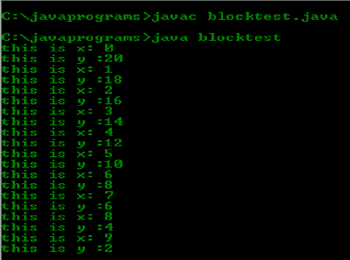## Write a program which contains block of code in “for” loop definition.

Category : Java | Sub Category : The Genesis of Java | By Prasad Bonam Last updated: 2020-09-28 13:28:37 Viewed : 378

## DESCRIPTION: in this we first declare a class as name blocktest, than we initialize x and y, and than we give value of y; after this we initialize for loop and then it give out put line which show x and y, and in program we declare value of y

Code:

/**

*

* Write a program which contains block of code in “for” loop definition.

*/

class blocktest { // this line declare class name

public static void main(String arg[]) { // this is the main line were program main funcation starts

int x, y; // in this line x and y is declaer as as int variable

y = 20; // in this line we give thie value of y

for (x = 0; x < 20; x++) { // this is a for loop that code run 20 time

System.out.println("this is x: " + x); // this line give us value of x 20 time

System.out.println("this is y :" + y); // this line give us value of y 20 time

y = y - 2;

} // this line show out put of y is given by this formula

}// this is the end of main

}// this is the end of class

OUTOUT: